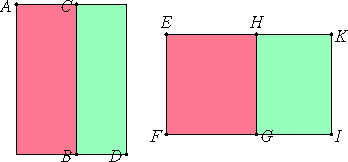# Proposition 71

If a rational and a medial are added together, then four irrational straight lines arise, namely a binomial or a first bimedial or a major or a side of a rational plus a medial area.

Let AB be rational, and CD medial.

I say that the side of the area AD is a binomial or a first bimedial or a major or a side of a rational plus a medial area.

For AB is either greater or less than CD.

First, let it be greater. Set out a rational straight line EF , apply to EF the rectangle EG equal to AB, producing EH as breadth, and apply to EF HI, equal to DC, producing HK as breadth.X.20

Then, since AB is rational and equals EG, therefore EG is also rational. And it is applied to EF, producing EH as breadth, therefore EH is rational and commensurable in length with EF.

X.22

Again, since CD is medial and equals HI, therefore HI is also medial. And it is applied to the rational straight line EF, producing HK as breadth, therefore HK is rational and incommensurable in length with EF.

Since CD is medial, while AB is rational, therefore AB is incommensurable with CD, so that EG is also incommensurable with HI.

But EG is to HI as EH is to HK, therefore EH is also incommensurable in length with HK.

X.36

And both are rational, therefore EH and HK are rational straight lines commensurable in square only. Therefore EK is a binomial straight line, divided at H.

Since AB is greater than CD, while AB equals EG and CD equals HI, therefore EG is also greater than HI. Therefore EH is also greater than HK. The square, then, on EH is greater than the square on HK either by the square on a straight line commensurable in length with EH or by the square on a straight line incommensurable with it.

First, let the square on it be greater by the square on a straight line commensurable with itself.

X.Def.II.1

Now the greater straight line HE is commensurable in length with the rational straight line EF set out, therefore EK is a first binomial.

X.54

But EF is rational, and, if an area is contained by a rational straight line and the first binomial, then the side of the square equal to the area is binomial. Therefore the side of EI is binomial, so that the side of AD is also binomial.

Next, let the square on EH be greater than the square on HK by the square on a straight line incommensurable with EH.

X.Def.II.4

Now the greater straight line EH is commensurable in length with the rational straight line EF set out, therefore EK is a fourth binomial.

X.57

But EF is rational, and, if an area be contained by a rational straight line and the fourth binomial, then the side of the area is the irrational straight line called major. Therefore the side of the area EI is major, so that the side of the area AD is also major.

Next, let AB be less than CD. Then EG is also less than HI, so that EH is also less than HK.

Now the square on HK is greater than the square on EH either by the square on a straight line commensurable with HK or by the square on a straight line incommensurable with it.

First, let the square on it be greater by the square on a straight line commensurable in length with itself.

X.Def.II.2

Now the lesser straight line EH is commensurable in length with the rational straight line EF set out, therefore EK is a second binomial.

X.55

But EF is rational, and, if an area is contained by a rational straight line and the second binomial, then the side of the square it is a first bimedial, therefore the side of the area EI is a first bimedial, so that the side of AD is also a first bimedial.

Next, let the square on HK be greater than the square on HE by the square on a straight line incommensurable with HK.

X.Def.II.5

Now the lesser straight line EH is commensurable with the rational straight line EF set out, therefore EK is a fifth binomial.

X.58

But EF is rational, and, if an area is contained by a rational straight line and the fifth binomial, then the side of the square equal to the area is a side of a rational plus a medial area.

Therefore the side of the area EI is a side of a rational plus a medial area, so that the side of the area AD is also a side of a rational plus a medial area.

Therefore, if a rational and a medial are added together, then four irrational straight lines arise, namely a binomial or a first bimedial or a major or a side of a rational plus a medial area.

Q.E.D.

## Guide

This proposition is not used in the rest of the Elements